# Maxwell-Boltzmann and Gamma

When I shared an image from the previous post on Twitter, someone who goes by the handle Nonetheless made the astute observation that image looked like the Maxwell-Boltzmann distribution. That made me wonder what 1/Γ(x) would be like turned into a probability distribution, and whether it would be approximately like the Maxwell-Boltzmann distribution.

(Here I’m looking at something related to what Nonetheless said, but different. He was looking at my plot of the error in approximating 1/Γ(x) by partial products, but I’m looking at 1/Γ(x) itself.)

## Making probability distributions

You can make any non-negative function with a finite integral into a probability density function by dividing it by its integral. So we can define a probability density functionwhereand so c = 0.356154. (The integral cannot be computed in closed form and so has to be evaluated numerically.)

Here’s a plot of f(x).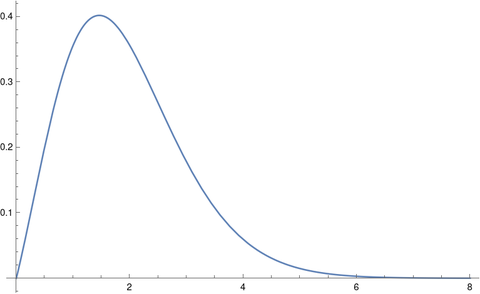Note that we’re doing something kind of odd here. It’s common to see the gamma function in the definition of probability distributions, but the function is always evaluated at distribution parameters, not at the free variable x. We’ll give an example of this shortly.

## Maxwell-Boltzmann distribution

The Maxwell-Boltzmann distribution, sometimes called just the Maxwell distribution, is used in statistical mechanics to give a density function for particle velocities. In statistical terminology, the Maxwell-Boltzmann distribution is a chi distribution  with three degrees of freedom and a scale parameter σ that depends on physical parameters.

The density function for a Maxwell-Boltzmann distribution with k degrees of freedom and scale parameter σ has the following equation.Think of this as xk-1 exp(-x² / 2σ²) multiplied by whatever you have to multiply it by to make it integrate to 1; most of the complexity in the definition is in the proportionality constant. And as mentioned above, the gamma function appears in the proportionality constant.

For the Maxwell-Boltzmann distribution, k = 3.

## Lining up distributions

Now suppose we want to compare the distribution we’ve created out of 1/Γ(x) with the Maxwell-Boltzman distribution. One way of to do this would be to align the two distributions to have their peaks at the same place. The mode of a chi random variable with k degrees of freedom and scale σ isand so for a given k we can solve for σ to put the mode where we’d like.

For positive values, the minimum of the gamma function, and hence the maximum of its reciprocal, occurs at 1.46163. As with the integral above, this has to be computed numerically.

For the Maxwell-Boltzmann distribution, i.e. when k = 3, we don’t get a very good fit.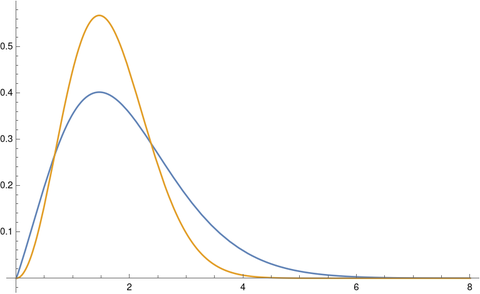The tails on the chi distribution are too thin. If we try again with k = 2 we get a much better fit.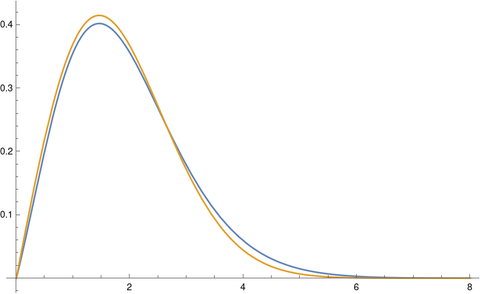You get an even better fit with k = 1.86. The optimal value of k would depend on your criteria for optimality, but k = 1.86 looks like a good value just eyeballing it.

 This is a chi distribution, not the far more common chi-square distribution. The first time you see this is looks like a typo. If X is a random variable with a chi random variable with k degrees of freedom then X² has a chi-square distribution with k degrees of freedom.

# Surprisingly not that surprisingWorld record marathon times have been falling in increments of roughly 30 seconds, each new record shaving roughly 30 seconds off the previous record. If someone were to set a new record, taking 20 seconds off the previous record, this would be exciting, but not suspicious. If someone were to take 5 minutes off the previous record, that would be suspicious.

One way to quantify how surprising a new record is would be to divide its margin of improvement over the previous margin of improvement. That is, given a new record y, and previous records y1 and y2, we can calculate an index of surprise by

r = (yy1) / (y1y2)

In  the authors analyze this statistic and extensions that take into account more than just the latest two records under technical assumptions I won’t get into here.

A p-value for the statistic R is given by

Prob(R > r) = 2/(r + 2).

You could think of this as a scale of surprise, with 0 being impossibly surprising and 1 being completely unremarkable.

There are multiple reasons to take this statistic with a grain of salt. It is an idealization based on assumptions that may not even approximately hold in a particular setting. And yet it is at least a useful rule of thumb.

The current marathon record beat the previous record by by 30 seconds. The previous margin of improvement was 78 seconds. This gives a value of r equal to 0.385 and a corresponding p-value of 0.84. This says the current record is impressive but statistically unremarkable. An improvement of 5 minutes, i.e. 300 seconds, would result in a p-value of 0.17, which is notable but not hard evidence cheating. 

The assumptions in  do not apply to marathon times, and may not apply to many situations where the statistic above nevertheless is a useful rule of thumb. The ideas in the paper could form the basis of a more appropriate analysis customized for a particular application.

Reports of a new record in any context are usually over-hyped. The rule of thumb above gives a way to gauge for yourself whether you should share the report’s excitement. You shouldn’t read too much into it, like any rule of thumb, but it at least gives a basis for deciding whether something deserves closer attention.

## More rule of thumb posts

 Andrew R. Solow and Woollcott Smith. How Surprising Is a New Record? The American Statistician, May, 2005, Vol. 59, No. 2, pp. 153-155

 I haven’t done the calculations, but I suspect that if you used the version of the index of surprise in  that takes into account more previous records, say the last 10, then you’d get a much smaller p-value.

The image at the top of the post is of Eliud Kipchoge, current marathon world record holder. Image under Creative Commons license. source.

# Big correlations and big interactions

An outcome cannot be highly correlated with a large number of independent predictors.

This observation has been called the piranha problem. Predictors are compared to piranha fish. If you have a lot of big piranhas in a small pond, they start eating each other. If you have a lot of strong predictors, they predict each other.

In  the authors quantify the piranha effect several ways. I’ll just quote the first one here. See the paper for several other theorems and commentary on their implications.

If X1, …, Xp, y are real-valued random variables with finite non-zero variance, thenSo if the left side is large, either because p is large or because some of the correlations are large, then the right side is also large, and so the sum of the interaction terms is large.

## Related posts

. The piranha problem: large effects swimming in a small pond. Available on arxiv.

# Design of experiments and design theoryDesign of experiments is a branch of statistics, and design theory is a branch of combinatorics, and yet they overlap quite a bit.

It’s hard to say precisely what design theory is, but it’s consider with whether objects can be arranged in certain ways, and if so how many ways this can be done. Design theory is pure mathematics, but it is of interest to people working in ares of applied mathematics such as coding theory and statistics.

Here’s a recap of posts I’ve written recently related to design of experiments and design theory.

## Design of Experiments

A few weeks ago I wrote about fractional factorial design. Then later I wrote about response surface models. Then a diagram from central composite design, a popular design in response surface methodology, was one the diagrams in a post I wrote about visually similar diagrams from separate areas of application.

I wrote two posts about pitfalls with A/B testing. One shows how play-the-winner sequential testing has the same problems as Condorcet’s voter paradox, with the order of the tests potentially determining the final winner. More seriously, A/B testing cannot detect interaction effects which may be critical.

## ANSI and Military Standards

There are several civilian and military standards related to design of experiments. The first of these was MIL-STD-105. The US military has retired this standard in favor of the civilian standard ASQ/ANSI Z1.4 which is virtually identical.

Similarly, the US military standard MIL-STD-414 was replaced by the very similar civilian standard ASQ/ANSI Z1.9. This post looks at the mean-range method for estimating variation which these two standards reference.

## Design Theory

I wrote a couple posts on Room squares, one on Room squares in general and one on Thomas Room’s original design now known as a Room square. Room squares are used in tournament designs.

I wrote a couple posts about Costas arrays, an introduction and a post on creating Costas arrays in Mathematica.

## Latin Squares

Latin squares and Greco-Latin squares a part of design theory and a part of design of experiments. Here are several posts on Latin and Greco-Latin squares.

# Three diagrams

This post will give examples of three similar diagrams that occur in three dissimilar areas: design of experiments, finite difference methods for PDEs, and numerical integration.

## Central Composite Design (CCD)

The most popular design for fitting a second-order response surface is the central composite design or CCD. When there are two factors being tested, the combinations of the two factors are chosen according to the following diagram, with the value at the center being used for several replications, say four.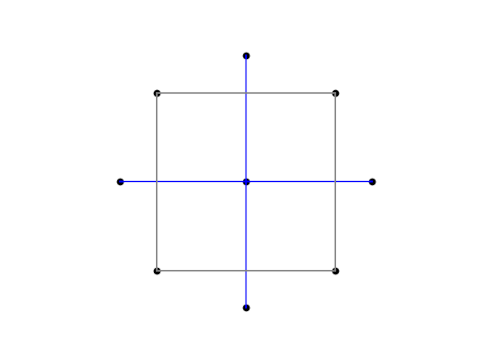The variables are coded so that the corners of the square have both coordinates equal to ±1. The points outside the square, the so-called axial points, have one coordinate equal to 0 and another equal to ±√2.

## Finite difference method

The star-like arrangement of points with the middle being weighted more heavily is reminiscent of the five-point finite difference template for the Laplacian. Notice that the center point is weighted by a factor of -4.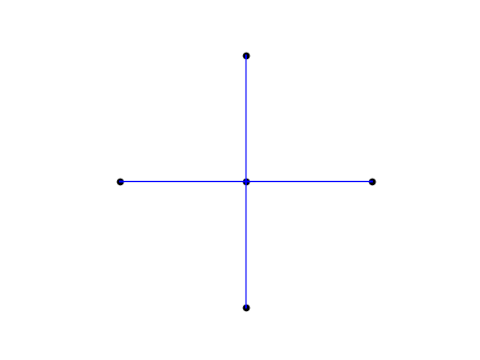In the finite difference method, this pattern of grid points carries over to a pattern of matrix coefficients.

## Integrating over a disk

The CCD grid looks even more like a numerical integration pattern for a function f defined over a disk of radius h. See A&S equation 25.4.61.The center of the circle has weight w = 1/6. The four points on the circle are located at (±h, 0) and (0, ±h) and have weight w = 1/24. The remaining points are located at (±h/2, ±h/2) and have weight w = 1/6.

# Another problem with A/B testing: interaction effectsThe previous post looked at a paradox with A/B testing: your final result may depend heavily on the order of your tests. This post looks at another problem with A/B testing: the inability to find interaction effects.

Suppose you’re debating between putting a photo of a car or a truck on your web site, and you’re debating between whether the vehicle should be red or blue. You decide to use A/B testing, so you test whether customers prefer a red truck or a blue truck. They prefer the blue truck. Then you test whether customers prefer a blue truck or a blue car. They prefer the blue truck.

Maybe customers would prefer a red car best of all, but you didn’t test that option. By testing vehicle type and color separately, you didn’t learn about the interaction of vehicle type and color. As Andrew Gelman and Jennifer Hill put it ,

Interactions can be important. In practice, inputs that have large main effects also tend to have large interactions with other inputs. (However, small main effects do not preclude the possibility of large interactions.)

Notice that sample size is not the issue. Suppose you tested the red truck against the blue truck with 1000 users and found that 88.2% preferred the blue truck. You can be quite confident that users prefer the blue truck to the red truck. Suppose you also used 1000 users to test the blue truck against the blue car and this time 73.5% preferred the blue truck. Again you can be confident in your results. But you failed to learn something that you might have learned if you’d split 100 users between four options: red truck, blue truck, red car, blue car.

## Experiment size

This is an example of a factorial design, testing all combinations of the factors involved. Factorial designs seem impractical because the number of combinations can grow very quickly as the number of factors increases. But if it’s not practical to test all combinations of 10 factors, for example, that doesn’t mean that it’s impractical to test all combinations of two factors, as in the example above. It is often practical to use a full factorial design for a moderate number of factors, and to use a fractional factorial design with more factors.

If you only test one factor at a time, you’re betting that interaction effects don’t matter. Maybe you’re right, and you can optimize your design by optimizing each variable separately. But if you’re wrong, you won’t know.

## Agility

The advantage of A/B tests is that they can often be done rapidly. Blue or red? Blue. Car or truck? Truck. Done. Now let’s test something else.

If the only options were between a rapid succession of tests of one factor at a time or one big, complicated statistical test of everything, speed might win. But there’s another possibility: a rapid succession of slightly more sophisticated tests.

Suppose you have 9 factors that you’re interested in, and you understandably don’t want to test several replications of 29 = 512 possibilities. You might start out with a (fractional) factorial design of 5 of the factors. Say that only one of these factors seems to make much difference, no matter what you pair it with. Next you do another experiment testing 5 factors at a time, the winner of the first experiment and the 4 factors you haven’t tested yet. This lets you do two small experiments rather than one big one.

Note that in this example you’re assuming that the factors that didn’t matter in the first experiment wouldn’t have important interactions with the factors in the second experiment. And your assumption might be wrong. But you’re making an educated guess, based on data from the first experiment. This is less than ideal, but it’s better than the alternative of testing every factor one at a time, assuming that no interactions matter. Assuming that some interactions don’t matter, based on data, is better than making a blanket assumption that no interactions matter, based on no data.

Testing more than one factor at a time can be efficient for screening as well as for finding interactions. It can help you narrow in on the variables you need to test more thoroughly.

## Related posts

 Andrew Gelman and Jennifer Hill. Data Analysis Using Regression and Multilevel/Hierarchical Models. Cambridge University Press, 2007.

# ASQ/ANSI Z1.4 sampling procedures

I mentioned the other day that the US military standard MIL-STD-105 for statistical sampling procedures lives on in the ASQ/ANSI standard Z1.4. The Department of Defense cancelled their own standard in 1995 in favor of adopting civilian standards, in particular ASQ/ANSI Z1.4.

There are two main differences between military standard and its replacement. First, the military standard is free and the ASNI standard costs \$199. Second, the ASNI standard has better typography. Otherwise the two standards are remarkably similar.

For example, the screen shot from MIL-STD-105 that I posted the other day appears verbatim in ASQ/ASNI Z1.4, except with better typesetting. The table even has the same name: “Table II-B Single sampling plans for tightened inspection (Master table).” Since the former is public domain and the latter is copyrighted, I’ll repeat my screenshot of the former.Everything I said about the substance of the military standard applies to the ASNI standard. The two give objective, checklist-friendly statistical sampling plans and acceptance criteria. The biggest strength and biggest weakness of these plans is the lack of nuance. One could create more sophisticated statistical designs that are more powerful, but then you lose the simplicity of a more regimented approach.

A company could choose to go down both paths, using more informative statistical models for internal use and reporting results from the tests dictated by standards. For example, a company could create a statistical model that takes more information into account and use it to assess the predictive probability of passing the tests required by sampling standards.

# Sharing data without letting it goSuppose two companies would like to share data, but they’d also each like to retain ownership of their own data. They’d like to enable querying as if each company had given the other all its data, without actually letting go of its data.

Maybe the two companies are competitors who want to collaborate for a particular project. Or maybe the companies each have data that they are not legally allowed to share with the other. Maybe one company is interested in buying (the data of) the other and would like to have some sort of preview of what they may be buying.

Differential privacy makes this possible, and can be useful even if privacy is not an issue. The two companies have data on inanimate widgets, not persons, and yet they have privacy-like concerns. They don’t want to hand over row-level data about their widgets, and yet they both want to be able to pose questions about the combined widget data. The situation is analogous to being concerned about the “privacy” of the widgets.

Both companies would deposit data with a trusted third party, and gain access to this data via an API that implements differential privacy. Such APIs let users pose queries but do not allow either side to request row-level data.

How is this possible? What if one party poses a query that unexpectedly turns out to be asking for row-level data? For example, maybe someone asks for the average net worth of customers in Alaska, assuming there are hundreds of such customers, but the data only contains one customer in Alaska. What was intended to be an aggregate query turns out to be a row-level query.

Differential privacy handles this sort of thing automatically. It adds an amount of random noise to each query in proportion to how sensitive the query is. If you ask for what amounts to data about an individual person (or individual widget) the system will add enough noise to the result to prevent revealing row-level information. (The system may also refuse to answer the query; this is done more often in practice than in theory.) But if you ask a question that reveals very little information about a single database row, the amount of noise added will be negligible.

The degree of collaboration can be limited up front by setting a privacy budget for each company. (Again, we may not necessarily be talking about the privacy of people. We may be looking at analogous protections on units of information about physical things, such as results of destructive testing of expensive hardware.)

Someone could estimate at the start of the collaboration how large the privacy budget would need to be to allow both companies to satisfy their objectives without being so large as to risk giving away intellectual property that the parties do not wish to exchange. This budget would be spent over the course of the project. When the parties exhaust their privacy budgets, they can discuss whether to allow each other more query budget.

This arrangement allows both parties the ability to ask questions of the combined data as if they had exchanged data. However, neither party has given up control of its data. They have given each other some level of information inferred from the combined data, but neither gives a single row of data to the other.

# Robustness of mean range

Let’s suppose we have data that comes from a distribution that is approximately normal but has a heavier right tail, specifically a gamma distribution with shape 6.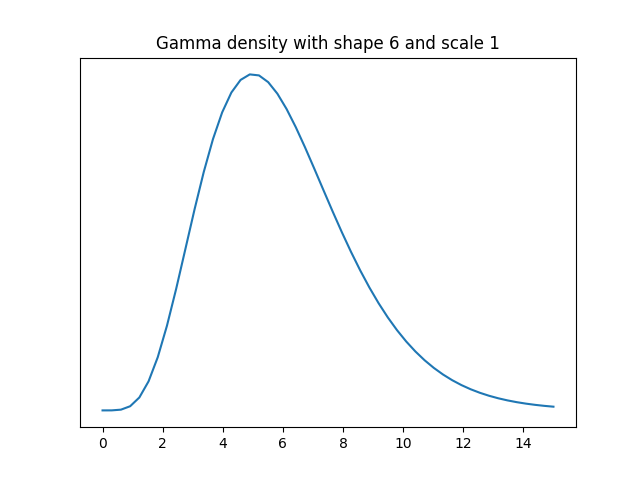We’d like to estimate the standard deviation of the data source. If the data were normally distributed, the sample standard deviation would be the most efficient unbiased estimator. But what about this example where the data are roughly normally distributed? How well does sample standard deviation compare to the mean range? Following the convention of ANSI Z1.4 we’ll average the ranges of samples of 5.

The variance of a gamma random variable with scale 1 is equal to its shape parameter, so our data has variance 6, and so standard deviation √6. We will pretend that we don’t know this and see how well we can infer this value using random samples. We’ll compare the statistical efficiency of the mean of the ranges of k samples of 5 each and the standard deviation based on 5k samples for k = 2 and 4.

First we’ll use the following code to estimate the population standard deviation with 10 samples two ways: with sample standard deviation and with the average of the range of two samples of 5. We’ll take the absolute value of the difference between each estimate and the true value, and repeat this 1,000 times to simulate the mean absolute error.

    from scipy.stats import gamma, t

def meanrange(y, k):
scale = 0.430 # scale factor for n=5
s = 0
for i in range(k):
sample = y[5*i:5*(i+1)]
s += max(sample) - min(sample)
return scale*s/k

n = 5
k = 2
shape = 6
sigma = shape**0.5

stderr = 0
mrerr = 0
N = 1000
for _ in range(N):
y = gamma(shape).rvs(size=n*k)
std = y.std(ddof=1)
mr = meanrange(y, k)
stderr += abs(std-sigma)
mrerr += abs(mr-sigma)
print(stderr/N, mrerr/N)


The mean absolute error was 0.532 using sample standard deviation and 0.550 using mean range.

When I changed k to 4, the mean absolute error using the sample standard deviation was 0.377. The mean absolute error using the range of 4 samples of 5 each time was 0.402.

The error in both methods was comparable, though the sample standard deviation did slightly better.

Next I repeated the experiment drawing samples from a Student t distribution with 3 degrees of freedom, a distribution with heavy tails.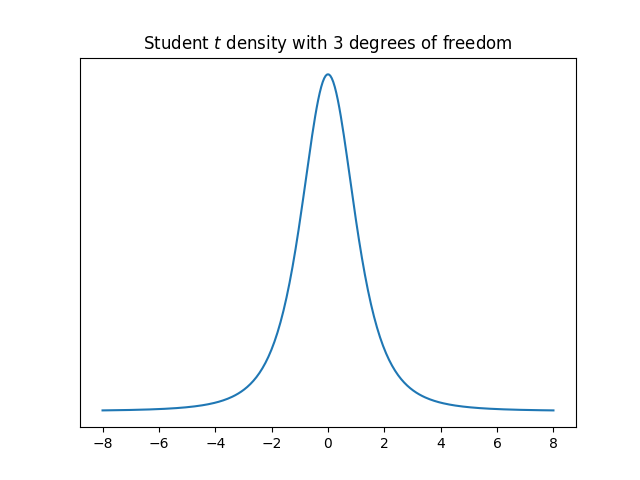When k = 2, the mean absolute errors were 0.603 and 0.574 for sample standard deviation and mean range respectively. When k = 4, the errors were 0.484 and 0.431. Again the errors are comparable, but this time the mean range was more accurate.

# Using mean range method to measure variability

The most common way to measure variability, at least for data coming from a normal distribution, is standard deviation. Another less common approach is to use mean range. Standard deviation is mathematically simple but operationally a little complicated. Mean range, on the other hand, is complicated to analyze mathematically but operationally very simple.

## ASQ/ANSI Z1.9

The ASQ/ANSI Z1.9 standard, Sampling Procedures and Table for Inspection by Variables for Percent Nonconforming, gives several options for measuring variability, and one of these is the mean range method. Specifically, several samples of five items each are drawn, and the average of the ranges is the variability estimate. The ANSI Z1.9 standard grew out of, and is still very similar to, the US military standard MIL-STD-414 from 1957. The ANSI standard, last updated in 2018, is not that different from the military standard from six decades earlier.

The average mean is obviously simple to carry out: take five samples, subtract the smallest value from the largest, and write that down. Repeat this a few times and average the numbers you wrote down. No squares, no square roots, easy to carry out manually. This was obviously a benefit in 1957, but not as much now that computers are ubiquitous. The more important advantage today is that the mean range can be more robust for heavy-tailed data. More on that here.

## Probability distribution

The distribution of the range of a sample is not simple to write down, even when the samples come from a normal distribution. There are nice asymptotic formulas for the range as the number of samples goes to infinity, but five is a bit far from infinity .

This is a problem that was thoroughly studied decades ago. The random variable obtained by averaging k ranges from samples of n elements each is denotedor sometimes without the subscripts.

## Approximate distribution

There are several useful approximations for the distribution of this statistic. Pearson  evaluated several proposed approximations and found that the best one for n < 10 (as it is in our case, with n = 5) to beHere σ is the standard deviation of the population being sampled, and the values of c and ν vary with n and k. For example, when n = 5 and k = 4, the value of ν is 14.7 and the value of c is 2.37. The value of c doesn’t change much as k gets larger, though the value of ν does .

Note that the approximating distribution is chi, not the more familiar chi square. (I won’t forget a bug I had to chase down years ago that was the result of seeing χ in a paper and reading it as χ². Double, triple, quadruple check, everything looks right. Oh wait …)

For particular values of n and k you could use the approximate distribution to find how to scale mean range to a comparable standard deviation, and to assess the relative efficiency of the two methods of measuring variation. The ANSI/ASQ Z1.9 standard gives tables for acceptance based on mean range, but doesn’t go into statistical details.

## Related posts

 Of course every finite number is far from infinity. But the behavior at some numbers is quite close to the behavior at infinity. Asymptotic estimates are not just an academic exercise. They can give very useful approximations for finite inputs—that’s why people study them—sometimes even for inputs as small as five.

 E. S. Pearson (1952) Comparison of two approximations to the distribution of the range in small samples from normal populations. Biometrika 39, 130–6.

 H. A. David (1970). Order Statistics. John Wiley and Sons.